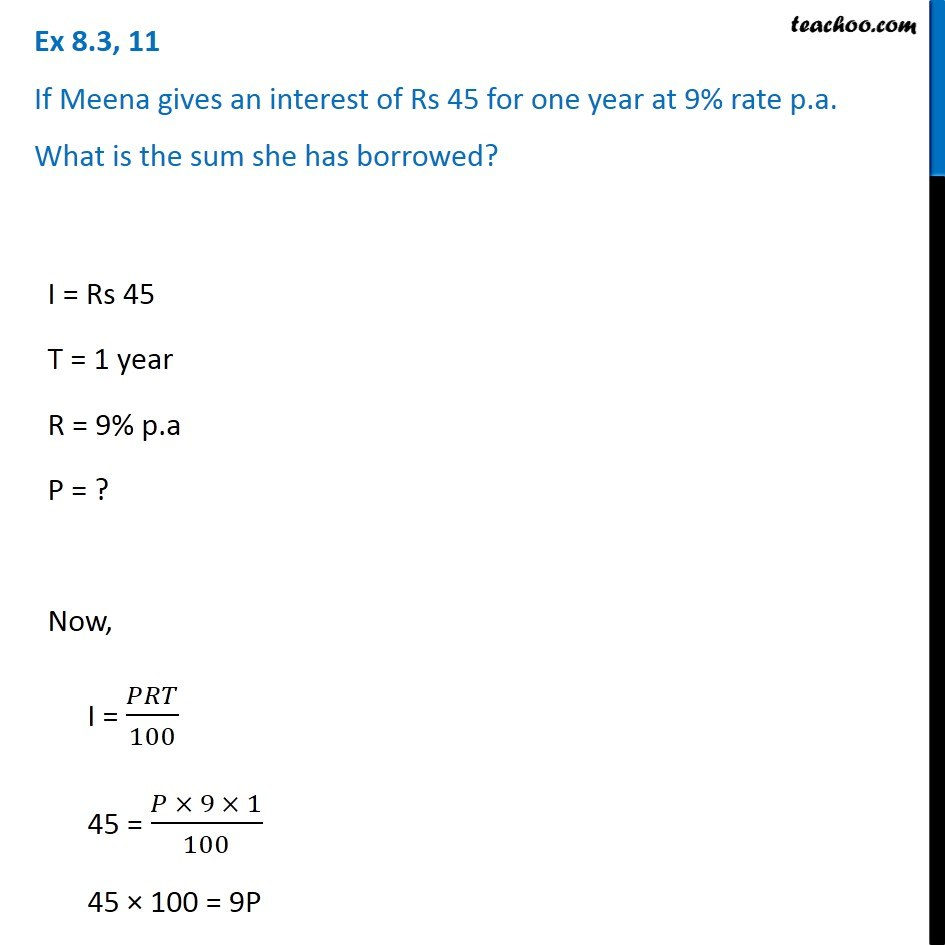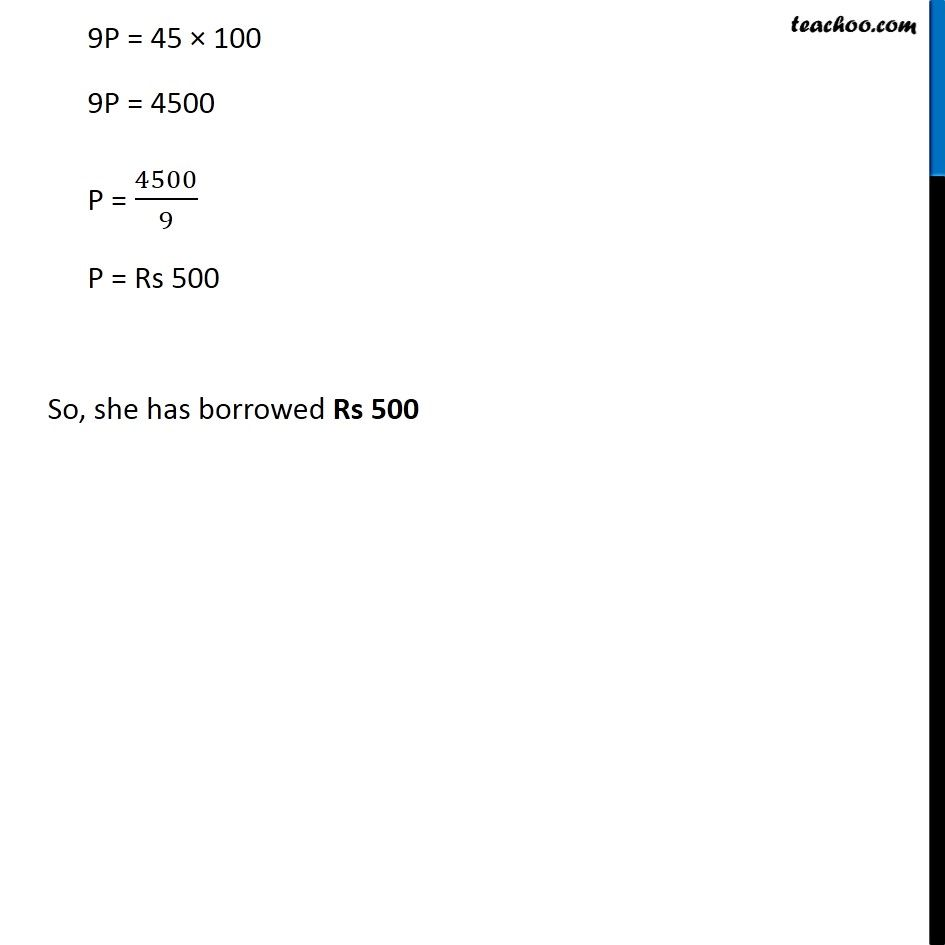Simple interest

Chapter 7 Class 7 Comparing Quantities
Concept wiseLearn in your speed, with individual attention - Teachoo Maths 1-on-1 Class

### Transcript

Ex 7.2, 11 If Meena gives an interest of Rs 45 for one year at 9% rate p.a. What is the sum she has borrowed? I = Rs 45 T = 1 year R = 9% p.a P = ? Now, I = 𝑃𝑅𝑇/100 45 = (𝑃 × 9 × 1)/100 45 × 100 = 9P 9P = 45 × 100 9P = 4500 P = 4500/9 P = Rs 500 So, she has borrowed Rs 500